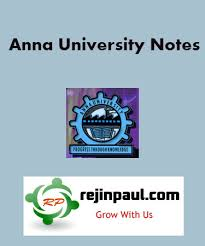### New Update

6/recent/ticker-posts

# MA8251 Engineering Mathematics II Syllabus Notes Question Paper Question Banks

## MA8251 Engineering Mathematics II Syllabus Notes Question Paper Question Banks Anna University - Regulation 2017 2nd Semester Notes CBCS

Anna University Engineering Mathematics II Syllabus Notes Question Bank Question Papers - MA8251 Syllabus Notes
Anna University MA8251 Engineering Mathematics II Notes is provided below. MA8251 Notes all 5 units notes are uploaded here. here MA8251 Maths 2 notes download link is provided and students can download the MA8251 Lecture Notes and can make use of it.MA8251 Engineering Mathematics II Syllabus Regulation 2017
UNIT I MATRICES
Eigenvalues and Eigenvectors of a real matrix – Characteristic equation – Properties of Eigenvalues and Eigenvectors – Cayley-Hamilton theorem – Diagonalization of matrices – Reduction of a quadratic form to canonical form by orthogonal transformation – Nature of quadratic forms.
UNIT II VECTOR CALCULUS
Gradient and directional derivative – Divergence and curl - Vector identities – Irrotational and Solenoidal vector fields – Line integral over a plane curve – Surface integral - Area of a curved surface - Volume integral - Green‘s, Gauss divergence and Stoke‘s theorems – Verification and application in evaluating line, surface and volume integrals.
UNIT III ANALYTIC FUNCTIONS
Analytic functions – Necessary and sufficient conditions for analyticity in Cartesian and polar coordinates - Properties – Harmonic conjugates – Construction of analytic function - Conformal mapping – Mapping by functions Bilinear transformation.
UNIT IV COMPLEX INTEGRATION
Line integral - Cauchy‘s integral theorem – Cauchy‘s integral formula – Taylor‘s and Laurent‘s series – Singularities – Residues – Residue theorem – Application of residue theorem for evaluation of real integrals – Use of circular contour and semicircular contour.
UNIT V LAPLACE TRANSFORMS

Existence conditions – Transforms of elementary functions – Transform of unit step function and unit impulse function – Basic properties – Shifting theorems -Transforms of derivatives and integrals – Initial and final value theorems – Inverse transforms – Convolution theorem – Transform of periodic functions – Application to solution of linear second order ordinary differential equations with constant coefficients.